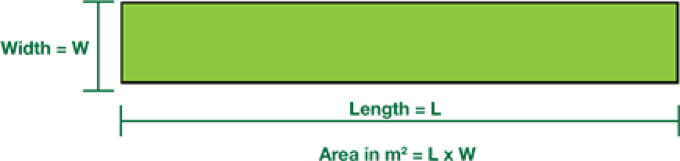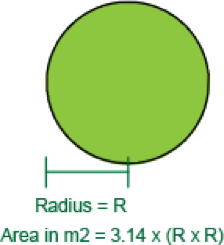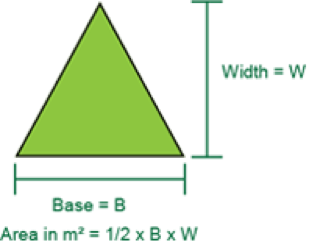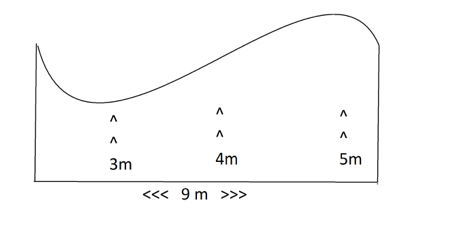Select Page# Measuring for Turf

## Measuring and ordering your turf

Turf measuring is an easy procedure that doesn’t require you to know advanced algebra. It’s simply calculating the ground area. But depending the size and shape of your block, it may get complex, which is why we have put together these examples below.

Our friendly team of experts are on hand to help you with your measurements so you get the right amount of turf for the job.

## How to calculate the turf required depending on the shape of the area:

##### SQUARE OR RECTANGLE
1. Measure the length (L) and width (W) of the square or rectangular area.
2. To calculate the amount of turf you need in square metres, multiply the length by the width.
3. For example: Length is 10m and width is 5m, so 10m x 5m = 50sqm.##### CIRCLE OR OVALCircle:

1. Measure the radius of the circular area.
2. For example: Radius = 4.5m, so 3.14 x 4.5m x 4.5m = 63.58sqm.

Oval:

1. Measure the length (L) and width (W) of the oval area. To calculate the amount of turf you need in square metres, multiply 0.80 by the length and then by the width.
2. For example: Length is 23m, width is 17m, so 0.80 x 23m x 17m = 80sqm.
##### TRIANGLE
1. Measure the base (B) and Width (W) of the triangular area. To calculate the amount of turf you need in square metres, multiply 0.50 by the base and then by the width.
2. For example: Base is 40m, width is 25m, so 0.50 x 40m x 25m = 500sqm.##### UNUSUAL SHAPES
1. First, divide the area into sections of regular shapes, for example, circle, square and triangle.
2. To calculate the amount of turf you need in square metres, apply formulas above for each shape.
##### DIFFERENT WIDTHS
1. First, measure the length of the longest axis across the area. Every 2m along the length line, measure the width of the area at right angles to the length line.
2. Total all widths, divide by the number of width section and multiply by the length.
3. For example: Length is 9m, width1 is 3m, width2 is 5m and width3 is 4m, so ((3m + 5m + 4m) ÷ 3) x 9m = 36sqm.NOTE: If the area is really curvy, you may need to add 5-7sq m/100sqm. If it is straight forward, add only a few extra square metres/100sqm.

Enquire with our friendly team for a sharp price on your turf today